# Kolm Index

## Definition

The Kolm Index' is a concentration index useful for the purpose of measuring name, sector or geographic concentration

## Details

If we have n exposures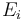$E_i$ summing up to a total exposure of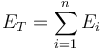$E_T = \sum^{n}_{i=1} E_{i}$

average exposure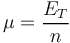$\mu = \frac{E_T}{n}$

and the fractional exposures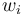$w_i$ are defined as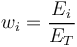$w_{i} = \frac{E_i}{E_T}$

Then the Kolm index is defined as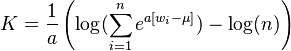$K = \frac{1}{a} \left( \log( \sum_{i=1}^n e^{ a [w_i - \mu]} ) - \log(n) \right)$

None

None

None

## Implementations

Open Source implementations of the Kolm index are available in